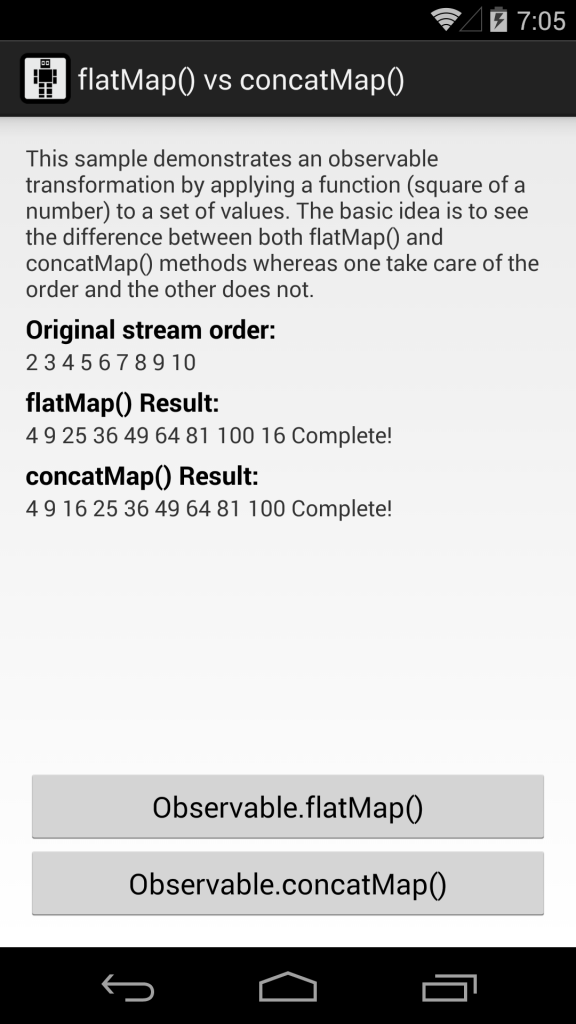# RxJava Observable tranformation: concatMap() vs flatMap().There are so many operators which transform streams of data. Using the right ones could be challenging but something we ought to, so let’s clear up the main difference between concatmap() and flatmap() in RxJava, which can save us a bit of headache.

“Programs must be written for people to read, and only incidentally for machines to execute.”

## Introduction

After a while I decided that was time to get back for some writing. As you may know at @SoundCloud we do a strong use of the reactive approach, but to be honest, I am not here to talk about RxJava itself because there are great articles out there to read about it (here and here) and great people to follow as well, such as Ben Christesen, Matthias Käppler and many others.

I also consider myself a “newbie” in reactive programming and now I am at that stage where you start seeing the benefits of this approach and want to make every single object reactive, which is very dangerous, so if you are in the same level as me, just keep an eye on it, and use it wherever makes sense, you are advised.

Let”s get started with the article then…

## Observable transformation

There are times where you have an Observable which you are subscribed to and you want to transform the results (remember that everything is a stream in Reactive Programming).

When it comes to observable transformation, the values from the sequences we consume are not always in the format or shape we need or each value needs to be expanded either into a richer object or into more values, so we can do this by applying a function to each element returned by your observable which will convert all of the items emitted by it into Observables and merge the result.

Do not worry if you do not understand yet (it took me a while to think the reactive way), we will see an example in a bit.

## The problem

I was retrieving a set of values from the database and applying a function to each of them that was suppose to both transform them in other objects asynchronously and also preserve their order.

Last step was to convert them into a list needed by the UI to display the results.

The behavior I had was not the expected one and here is why: I was using Observable.flatMap() which does not preserve the order of the elements.

## A simple example

Let me put a simple example to demonstrate the mentioned behavior.

Let”s say we have an Observable emitting a set of Integers and we want to calculate the square of each of those values:

public class DataManager {
private final List<Integer> numbers;
private final Executor jobExecutor;

public DataManager() {
this.numbers = new ArrayList<>(Arrays.asList(2, 3, 4, 5, 6, 7, 8, 9, 10));
jobExecutor = JobExecutor.getInstance();
}

public Observable<Integer> getNumbers() {
return Observable.from(numbers);
}

return this.numbers;
}

public Observable<Integer> squareOf(int number) {
return Observable.just(number * number).subscribeOn(Schedulers.from(this.jobExecutor));
}
}


Here our DataManager class has a method that returns an Observable which emits numbers from 2 to 10.

Then we want to calculate the square of those values so here is our function to apply to each of them:

private final Func1<Integer, Observable<Integer>> SQUARE_OF_NUMBER =
new Func1<Integer, Observable<Integer>>() {
@Override public Observable<Integer> call(Integer number) {
return dataManager.squareOf(number);
}
};


This will take an Integer as entry, will generate an Observable<Integer>, merge them and emit the results.

As you can see we are using a call to dataManager.squareOf() method which is asynchronous (for demonstration purpose) and looks something like this:

public Observable<Integer> squareOf(int number) {
return Observable.just(number * number).subscribeOn(Schedulers.from(this.jobExecutor));
}


Of course this works, but not as expected (at least the way I wanted), the order of the elements is not preserved (logcat output):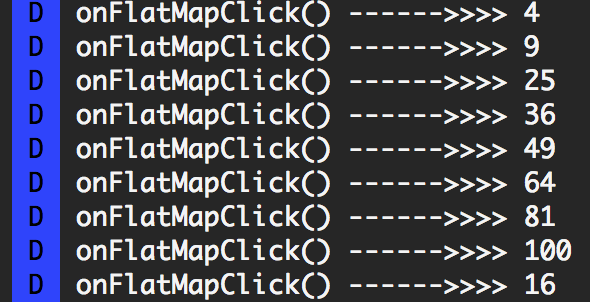## flatMap() vs concatMap()

Both methods look pretty much the same, but there is a difference: operator usage when merging the final results.

Here is some stuff from the official documentation: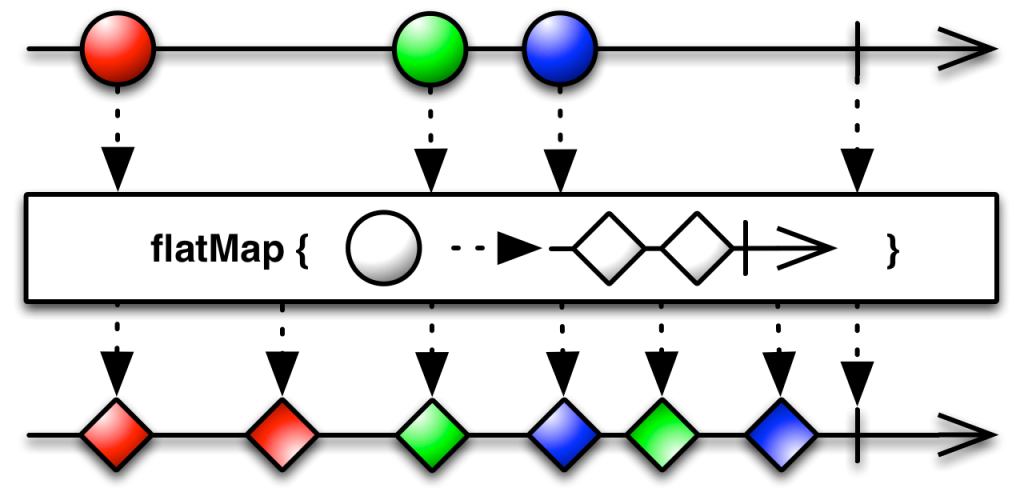DEFINITION: The flatMap() method creates a new Observable by applying a function that you supply to each item emitted by the original Observable, where that function is itself an Observable that emits items, and then merges the results of that function applied to every item emitted by the original Observable, emitting these merged results.

Note that flatMap() may interleave the items emitted by the Observables that result from transforming the items emitted by the source Observable.

If it is important that these items not be interleaved, you can instead use the similar concatMap() method.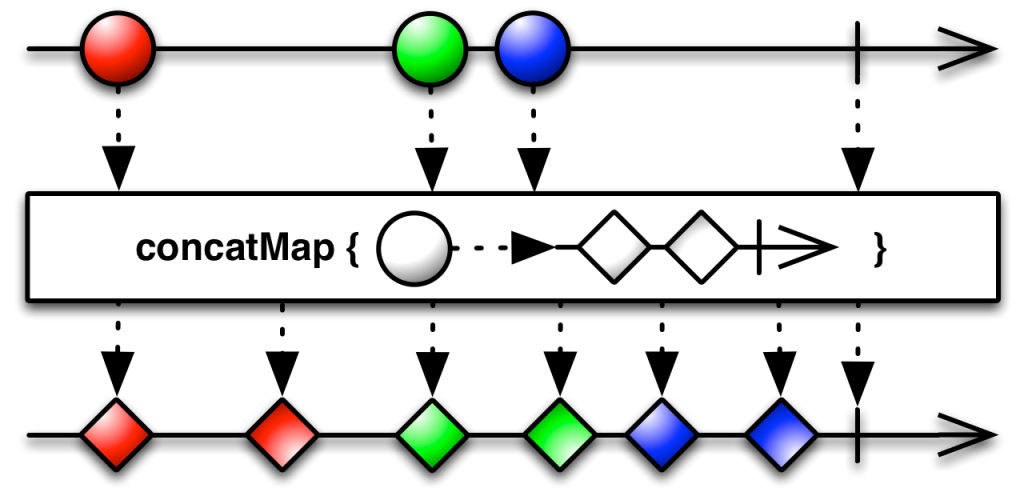As you can see, the two functions are very similar and the subtle difference is how the output is created (after the mapping function is applied . flatMap() uses MERGE operator while concatMap() uses CONCAT operator meaning that the last one cares about the order of the elements, so keep an eye on that if you need ordering :).

## Merge operator

Combine multiple Observables into one.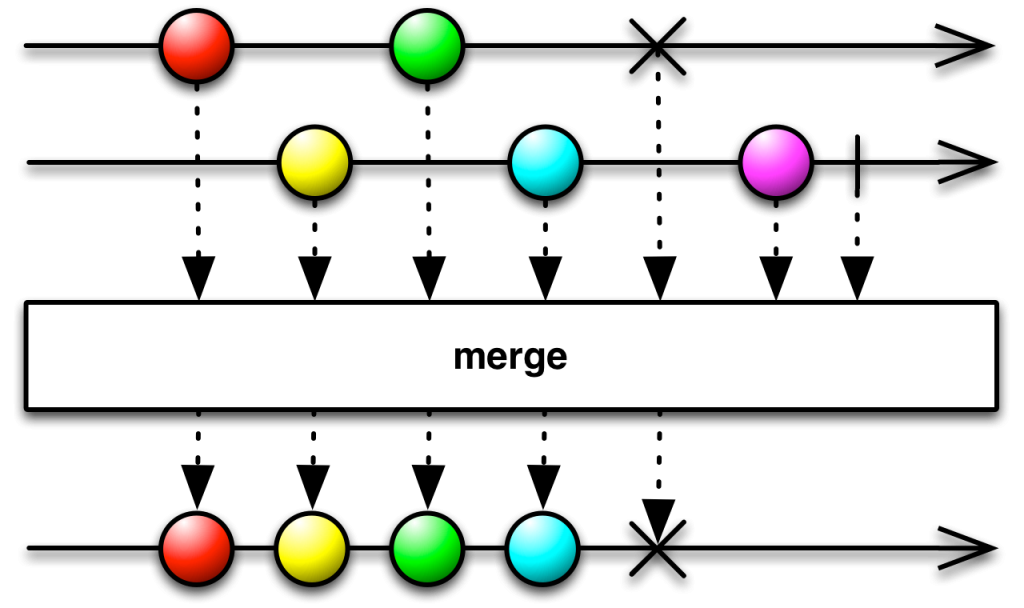## Concat operator

Concatenate two or more Observables sequentially.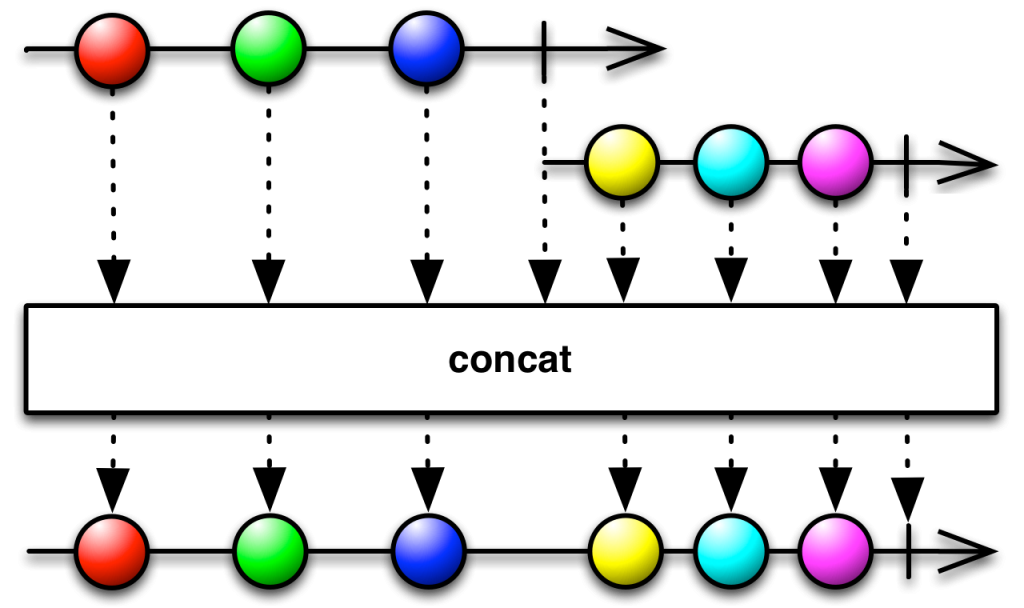## Problem solved

Observable concatMap() for the salvation! The problem was easily solved by just switching to a concatMap() method.

I know you may argue why I did not read the documentation first, which is very well explained by the way (kudos to the RxJava contributors!!!), but sometimes we are lazy or that is the last place we look into.

Here is a picture with the final results and some test I did (you can find the sample code below):